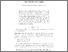# Measuring club-sequences together with the continuum large

Asperó, David and Mota, Miguel Angel (2017) Measuring club-sequences together with the continuum large. Journal of Symbolic Logic, 82 (3). pp. 1066-1079. ISSN 0022-4812Preview PDF (Accepted manuscript) - Accepted Version Download (394kB) | Preview

## Abstract

Measuring says that for every sequence $(C_\delta)_{\delta<\omega_1}$ with each $C_\delta$ being a closed subset of $\delta$ there is a club $C\subseteq\omega_1$ such that for every $\delta\in C$,a tail of $C\cap\delta$ is either contained in or disjoint from $C_\delta$. We answer a question of Justin Moore by building a forcing extension satisfying measuring together with $2^{\aleph_0}>\aleph_2$. The construction works over any model of ZFC + CH and can be described as a finite support forcing iteration with systems of countable models as side conditions and with symmetry constraints imposed on its initial segments. One interesting feature of this iteration is that it adds dominating functions $f:\omega_1\longrightarrow\omega_1$ mod. countable at each of its stages.

Item Type: Article measuring,large continuum,iterated forcing with symmetric systems of models as side conditions Faculty of Science > School of Mathematics Faculty of Science > Research Groups > Logic Pure Connector 13 Jan 2017 00:05 22 Oct 2022 02:05 https://ueaeprints.uea.ac.uk/id/eprint/62026 10.1017/jsl.2017.4View Item